Test: Circuit Analysis in the S-Domain

# Test: Circuit Analysis in the S-Domain

Test Description

## 20 Questions MCQ Test GATE Electrical Engineering (EE) 2023 Mock Test Series | Test: Circuit Analysis in the S-Domain

Test: Circuit Analysis in the S-Domain for Railways 2023 is part of GATE Electrical Engineering (EE) 2023 Mock Test Series preparation. The Test: Circuit Analysis in the S-Domain questions and answers have been prepared according to the Railways exam syllabus.The Test: Circuit Analysis in the S-Domain MCQs are made for Railways 2023 Exam. Find important definitions, questions, notes, meanings, examples, exercises, MCQs and online tests for Test: Circuit Analysis in the S-Domain below.
Solutions of Test: Circuit Analysis in the S-Domain questions in English are available as part of our GATE Electrical Engineering (EE) 2023 Mock Test Series for Railways & Test: Circuit Analysis in the S-Domain solutions in Hindi for GATE Electrical Engineering (EE) 2023 Mock Test Series course. Download more important topics, notes, lectures and mock test series for Railways Exam by signing up for free. Attempt Test: Circuit Analysis in the S-Domain | 20 questions in 60 minutes | Mock test for Railways preparation | Free important questions MCQ to study GATE Electrical Engineering (EE) 2023 Mock Test Series for Railways Exam | Download free PDF with solutions
 1 Crore+ students have signed up on EduRev. Have you?
Test: Circuit Analysis in the S-Domain - Question 1

### The ratio of the transfer function Io/Is is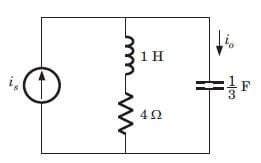Detailed Solution for Test: Circuit Analysis in the S-Domain - Question 1

Io/Is = (s + 4)/ (s + 4 + 3/s) = s(s + 4)/(s + 1)(s + 3).

Test: Circuit Analysis in the S-Domain - Question 2

### The voltage across 200 μF capacitor is given by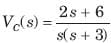The steady state voltage across capacitor is

Detailed Solution for Test: Circuit Analysis in the S-Domain - Question 2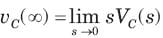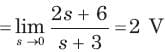Test: Circuit Analysis in the S-Domain - Question 3

### The transformed voltage across the 60 μF capacitor is given by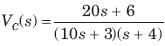The initial current through capacitor is

Detailed Solution for Test: Circuit Analysis in the S-Domain - Question 3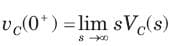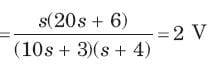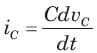⇒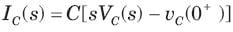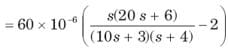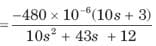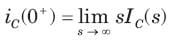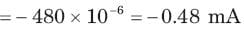Test: Circuit Analysis in the S-Domain - Question 4

The current through an 4 H inductor is given by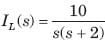The initial voltage across inductor is

Detailed Solution for Test: Circuit Analysis in the S-Domain - Question 4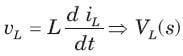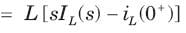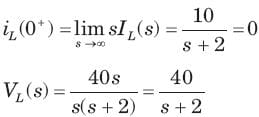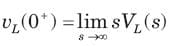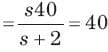Test: Circuit Analysis in the S-Domain - Question 5

The amplifier network shown in fig.is stable if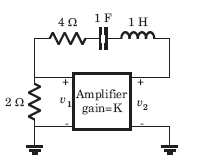Detailed Solution for Test: Circuit Analysis in the S-Domain - Question 5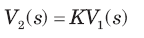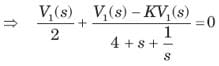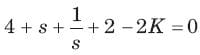⇒ s2 + (6 - 2K) s + 1 = 0
(6 - 2K) > 0  ⇒ K < 3

Test: Circuit Analysis in the S-Domain - Question 6

The network shown in fig. is stable if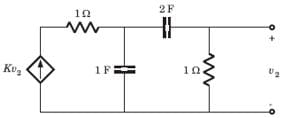Detailed Solution for Test: Circuit Analysis in the S-Domain - Question 6

Let v1 be the node voltage of middle node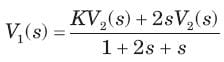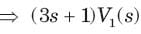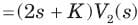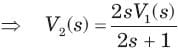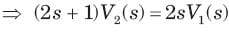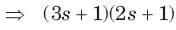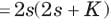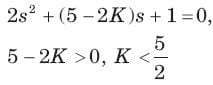Test: Circuit Analysis in the S-Domain - Question 7

A circuit has a transfer function with a pole s = -4 and a zero which may be adjusted in position as s= -a The response of this system to a step input has a term of form Ke -4t. The K will be (H= scale factor)

Detailed Solution for Test: Circuit Analysis in the S-Domain - Question 7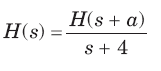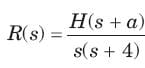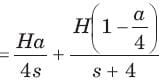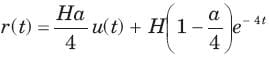Test: Circuit Analysis in the S-Domain - Question 8

A circuit has input vin (t) = cos 2t u(t) V and output io (t) = 2sin 2t u(t) A. The circuit had no internal stored energy at t = 0. The admittance transfer function is

Detailed Solution for Test: Circuit Analysis in the S-Domain - Question 8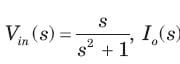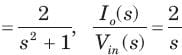Test: Circuit Analysis in the S-Domain - Question 9

A two terminal network consists of a coil having an inductance L and resistance R shunted by a capacitor C. The poles of the driving point impedance function Z of this network are at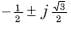and zero at -1. If Z(0) =1the value of R, L, C are

Detailed Solution for Test: Circuit Analysis in the S-Domain - Question 9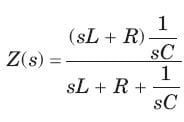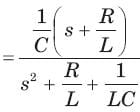Test: Circuit Analysis in the S-Domain - Question 10

The current response of a network to a unit step input is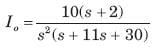The response is

Detailed Solution for Test: Circuit Analysis in the S-Domain - Question 10

The characteristic equation is  s2 (s2 + 11s + 30) = 0 ⇒ s2 (s + 6)(s + 5) = 0
s = -6, -5, Being real and unequal, it is overdamped.

Test: Circuit Analysis in the S-Domain - Question 11

The circuit is shown in fig.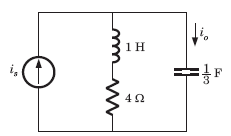The current ratio transfer function Io/IS  IS

Detailed Solution for Test: Circuit Analysis in the S-Domain - Question 11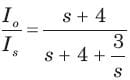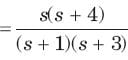Test: Circuit Analysis in the S-Domain - Question 12

The circuit is shown in fig.The response is

Detailed Solution for Test: Circuit Analysis in the S-Domain - Question 12

The characteristic equation is (s+1 (s+3) = 0. Being real and unequal root, it is overdamped response.

Test: Circuit Analysis in the S-Domain - Question 13

The circuit is shown in fig.If input is is 2u(t) A, the output current io is

Detailed Solution for Test: Circuit Analysis in the S-Domain - Question 13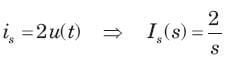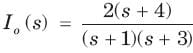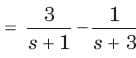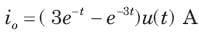Test: Circuit Analysis in the S-Domain - Question 14

In the network of Fig. , all initial condition are zero. The damping exhibited by the network is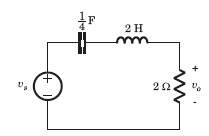Detailed Solution for Test: Circuit Analysis in the S-Domain - Question 14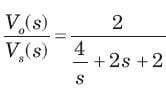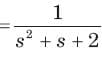The roots are imaginary so network is underdamped

Test: Circuit Analysis in the S-Domain - Question 15

The voltage response of a network to a unit step input is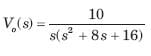The response is

Detailed Solution for Test: Circuit Analysis in the S-Domain - Question 15

The characteristic equation is s(s2 + 8s + 16) = 0 , (s + 4)2 = 0, s = -4, -4

Being real and repeated root, it is critically damped.

Test: Circuit Analysis in the S-Domain - Question 16

The response of an initially relaxed circuit to a signal vs is e-2t u(t). If the signal is changed to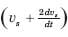, the response would be

Detailed Solution for Test: Circuit Analysis in the S-Domain - Question 16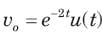⇒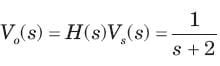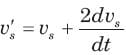⇒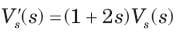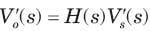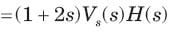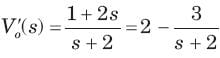⇒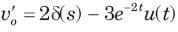Test: Circuit Analysis in the S-Domain - Question 17

Consider the following statements in the circuit shown in fig.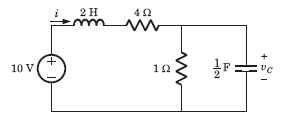1. It is a first order circuit with steady state value of vC= 10/3, i = 5/3A

2. It is a second order circuit with steady state of v= 2 V , i = 2 A

3. The network function V(s)/I(s)has one pole.

4. The network function V(s)/I(s) has two poles.

Detailed Solution for Test: Circuit Analysis in the S-Domain - Question 17

It is a second order circuit. In steady state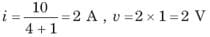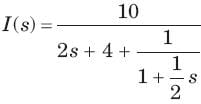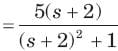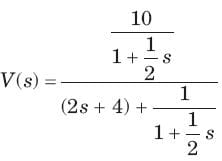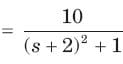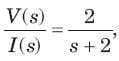It has one pole at s = -2

Test: Circuit Analysis in the S-Domain - Question 18

The network function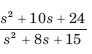represent a

Detailed Solution for Test: Circuit Analysis in the S-Domain - Question 18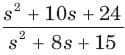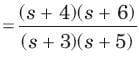The singularity near to origin is pole. So it may be RC impedance or RL admittance function.

Test: Circuit Analysis in the S-Domain - Question 19

The network function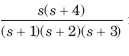represents an

Detailed Solution for Test: Circuit Analysis in the S-Domain - Question 19

Poles and zero does not interlace on negative real axis so it is not a immittance function

Test: Circuit Analysis in the S-Domain - Question 20

The network function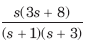represents an

Detailed Solution for Test: Circuit Analysis in the S-Domain - Question 20

The singularity nearest to origin is a zero. So it may be RL impedance or RC admittance function. Because of (D) option it is required to check that it is a valid RC admittance function. The poles and zeros interlace along the negative real axis. The residues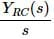are real and positive.

## GATE Electrical Engineering (EE) 2023 Mock Test Series

22 docs|274 tests
Information about Test: Circuit Analysis in the S-Domain Page
In this test you can find the Exam questions for Test: Circuit Analysis in the S-Domain solved & explained in the simplest way possible. Besides giving Questions and answers for Test: Circuit Analysis in the S-Domain, EduRev gives you an ample number of Online tests for practice

## GATE Electrical Engineering (EE) 2023 Mock Test Series

22 docs|274 tests(Scan QR code)